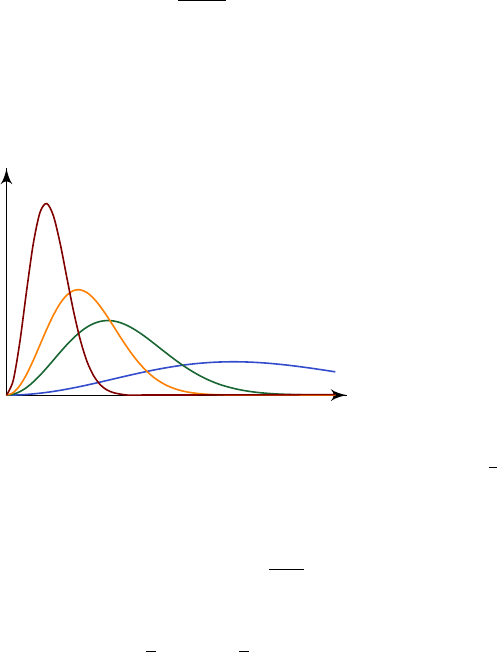2Classical gases

II Statistical Physics2.3 Maxwell distribution
We calculated somewhere the average energy of our gas, so we can calculate
the average energy of an atom in the gas. But that is just the average energy.
We might want to figure out the distribution of energy in the atoms of our gas.
Alternatively, what is the distribution of particle speed in a gas?
We can get that fairly straightforwardly from what we’ve got so far.
We ask what’s the probability of a given particle being in a region of phase
space of volume d
3
q d
3
p centered at (q, p). We know what this is. It is just
Ce
βp
2
/2m
d
3
q d
3
p
for some normalization constant
C
, since the kinetic energy of a particle is
p
2
/
2
m
. Now suppose we don’t care about the position, and just want to know
about the momentum. So we integrate over
q
, and the probability that the
momentum is within d
3
p of p is
CV d
3
p e
βp
2
/2m
.
Let’s say we are interested in velocity instead of momentum, so this is equal to
CV m
2
d
3
v e
βmv
2
/2
.
Moreover, we are only interested in the speed, not the velocity itself. So we
introduce spherical polar coordinates (v, θ, φ) for v. Then we get
CV m
3
sin θ dθ dϕ v
2
dv e
mv
2
/(2kT )
.
Now we don’t care about the direction, so we again integrate over all possible
values of θ and φ. Thus, the probability that the speed is within dv of v is
f(v) dv = Nv
2
e
mv
2
/(2kT )
dv,
where we absorbed all our numbers into the constant
N
. Then
f
is the probability
density function of v. We can fix this constant N by normalizing:
Z
0
f(v) dv = 1.
So this fixes
N = 4π
m
2πkT
1/2
.
This f(v) is called the Maxwell distribution.
We can try to see what
f
looks like. We see that for large
v
, it is exponentially
decreasing, and for small
v
v
. We can plot it for a few
monoatomic ideal gases:
T
C
4
He
20
Ne
40
Ar
132
Xe
We see that the energy distribution shifts to the right as we increase the mass.
This is expected, because we know that the energy of the particle is always
1
2
kT
,
and so for lighter particles, we need to have higher energy.
We can sanity-check that the expected value of v is correct. We have
hv
2
i =
Z
0
v
2
f(v) dv =
3kT
m
.
So we find that
hEi =
1
2
mhv
2
i =
3
2
kT.
This agrees with the equipartition of energy, as it must. But now we have an
actual distribution, we can compute other quantities like hv
4
i.
Note that in these derivations, we assumed we were dealing with a monoatomic
ideal gas, but it happens that in fact this holds for a much more general family
of gases. We will not go much into details.